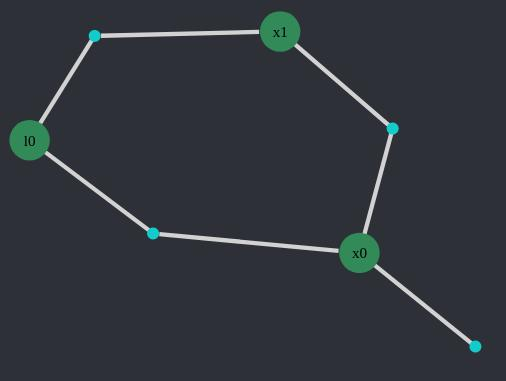Building Graphs

# Building Graphs

In this section constructing DFG graphs will be discussed. To start, bring DistributedFactorGraphs into your workspace:

``using DistributedFactorGraphs``

We recommend using IncrementalInference (IIF) to populate DFG graphs. DFG provides the structure, but IIF overloads the provided `addVariable!` and `addFactor!` functions and creates solver-specific data that allows the graph to be solved. So although you can use DFG's `addVariable!` and `addFactor!`, it is better to start with IIF's functions so that the graph is solvable.

So for the following examples, IncrementalInference will be used to create the variables and factors. It should be added and imported to run the examples:

``````using Pkg
``using IncrementalInference``

## Initializing a Graph

DFG graphs can be built using various drivers (different representations of the underlying graph). At the moment DFG supports 2 drivers:

• LightDFG: An in-memory graph that uses LightGraphs.jl for representing the graph.
• CloudGraphs: A database-driven graph that uses Neo4j.jl for interacting with the graph.

In general the in-memory drivers are used for building and solving graphs, and CloudGraphs is used for persisting in-memory graphs into a database.

To continue the example, run one of the following to create a DFG driver:

### Creating a LightDFG Graph

``````# Create a DFG with default solver parameters using the LightGraphs.jl driver.
dfg = LightDFG{SolverParams}(solverParams=SolverParams())``````

### Creating a CloudGraphsDFG Graph

``````# Create a DFG with no solver parameters (just to demonstrate the difference) using the CloudGraphs driver, and connect it to a local Neo4j instance.
cfg = CloudGraphsDFG{NoSolverParams}("localhost", 7474, "neo4j", "test",
"testUser", "testRobot", "testSession")``````

## Creating Variables and Factors

DFG and IIF rely on a CRUD (Create, Read, Update, and Delete) interface to allow users to create and edit graphs.

### Creating Variables with IIF

Variables are added using IncrementalInference's `addVariable!` function. To create the variable, you provide the following parameters:

• The graph the variable is being added to
• The variable's label (e.g. :x1 or :a)
• The variable inference type (aka soft type), which is a subtype of InferenceVariable

NOTE: Once variables are initialized to a specific soft type, variable node data (solver data) is templated to that type.

In addition, the following optional parameters are provided:

• Additional labels for the variable (in DFG these are referred to as tags)
• A `solvable` flag to indicate whether the variable is ready to be added to a solution

``````v1 = addVariable!(dfg, :x0, ContinuousScalar, tags = [:POSE], solvable=1)
v2 = addVariable!(dfg, :x1, ContinuousScalar, tags = [:POSE], solvable=1)
v3 = addVariable!(dfg, :l0, ContinuousScalar, tags = [:LANDMARK], solvable=1)``````

### Creating Factors with IIF

Similarly to variables, it is recommended that users start with the IIF implementation of the `addFactor!` functions to create factors. To create the factors, you provide the following parameters:

• The graph the variable is being added to
• The labels for the variables that the factor is linking
• The factor function (which is a subtype of )

Additionally, the solvable flag is also set to indicate that the factor can be used in solving graphs.

NOTE: Every graph requires a prior for it to be solvable, so it is a good practice to make sure one is added (generally by adding to the first variable in the graph).

Four factors are added: a prior, a linear conditional relationship with a normal distribution between x0 and x1, and a pair of linear conditional relationships between each pose and the landmark.

``````prior = addFactor!(dfg, [:x0], Prior(Normal(0,1)))
f1 = addFactor!(dfg, [:x0; :x1], LinearConditional(Normal(50.0,2.0)), solvable=1)
f1 = addFactor!(dfg, [:l0; :x0], LinearConditional(Normal(40.0,5.0)), solvable=1)
f1 = addFactor!(dfg, [:l0; :x1], LinearConditional(Normal(-10.0,5.0)), solvable=1)``````

The produced factor graph is:## Listing Variables and Factors

Reading, updating, and deleting all use DFG functions (as opposed to adding, where using the IncrementalInference functions are recommended).

Each variable and factor is uniquely identified by its label. The list of variable and factor labels can be retrieved with the `ls`/`listVariables` and `lsf`/`listFactors` functions:

For example listing the variables in the graph we created above:

``ls(dfg)``

Or listing the factors:

``lsf(dfg)``
``````1-element Vector{Symbol}:
:x0f1``````

To list all variables or factors (instead of just their labels), use the `getVariables` and `getFactors` functions:

Traversing and Querying functions for finding the relationships and building subtraphs include:

## Getting (Reading) Variables and Factors

Individual variables and factors can be retrieved from their labels using the following functions:

It is worth noting that `getVariable` allows a user to retrieve only a single solver entry, so that subsets of the solver data can be retrieved individually (say, in the case that there are many solutions). These can then be updated independently using the functions as discussed in the update section below.

## Updating Variables and Factors

Full variables and factors can be updated using the following functions:

NOTE: Skeleton and summary variables are read-only. To perform updates you should use the full factors and variables.

NOTE: `updateVariable`/`updateFactor` performs a complete update of the respective node. It's not a very efficient way to edit fine-grain detail. There are other methods to perform smaller in-place changes. This is discussed in more detail in Data Structure.

## Deleting Variables and Factors

Variables and factors can be deleted using the following functions: